﻿ 日照海岸带动力过程与地貌特征分析

日照海岸带动力过程与地貌特征分析Analysis of Dynamic Process and Geomorphologic Characteristics of Coastal Zone in Rizhao

Abstract: As a famous coastal city, Rizhao has the unique geographical location that makes it have very rich marine resource and coastal tourism resources. Rizhao Coastal Zone is as the research object in this article. A field survey was carried out on the coastal zone of Rizhao City and sample collection was performed in typical areas. The dynamic process and geomorphological characteristics of the Rizhao Coastal Zone are studied. The sediment grain size, hydrodynamic process and geomorphologic characteristics of Rizhao coastal zone are analyzed by the experiment of the samples and the experimental data. The following conclusions are drawn: The average diameter of the survey beaches in the coastal zone of Rizhao is between 0.7 φ and 2.5 φ. In general, the sorting of the beach is medium or relatively poor, the grain size frequency curve is sharp, the kurtosis is mainly distributed in a narrow state, and the skew state is mainly a negative bias state. The types of beaches in the coastal area of Rizhao City are mainly sand bar-lagoon type and straight type. The important area of Wanpingkou bathing beach is sand bar-lagoon type. The results of this study will help to understand the development and utilization of the current Rizhao coastal zone and provide credible data support and theoretical basis for the researchers to analyze the zone of Rizhao City and instruct the government development and utilization in the zone of Rizhao City.

1. 引言

2. 研究区概况

3. 研究方法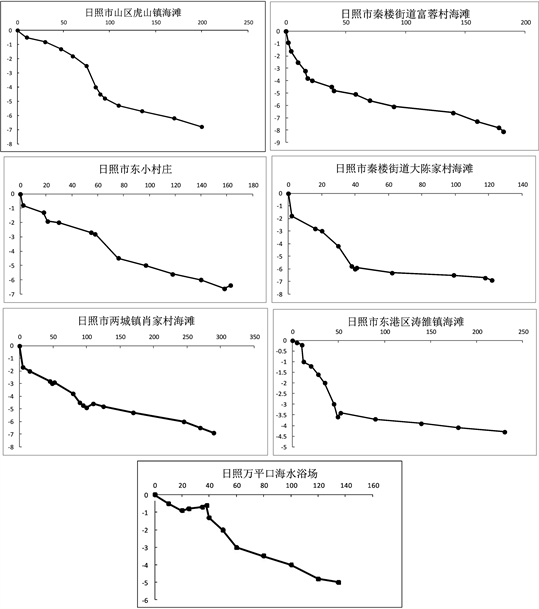Figure 1. Research area beach profile

4. 结果与分析

4.1. 海滩粒度分析

${M}_{Z}=\frac{\left({\phi }_{16}+{\phi }_{50}+{\phi }_{84}\right)}{3}$ (1)

$\sigma =\frac{\left({\phi }_{84}-{\phi }_{16}\right)}{4}+\frac{\left({\phi }_{95}-{\phi }_{5}\right)}{6.6}$ (2)

$SK=\frac{\left({\phi }_{16}+{\phi }_{84}-2{\phi }_{50}\right)}{2\left({\phi }_{84}-{\phi }_{16}\right)}+\frac{\left({\phi }_{5}+{\phi }_{95}-2{\phi }_{50}\right)}{2\left({\phi }_{95}-{\phi }_{5}\right)}$ (3)

$Kg=\frac{{\phi }_{95}-{\phi }_{5}}{2.44\left({\phi }_{75}-{\phi }_{25}\right)}$ (4)Table 2. Beach sediment particle size parameter table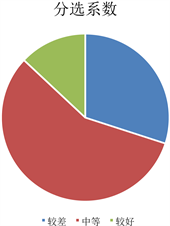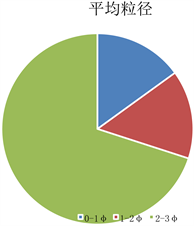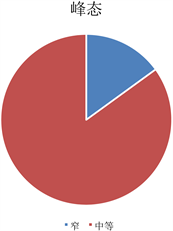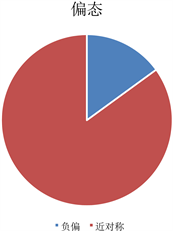Figure 2. Beach sediment particle size ratio in the study area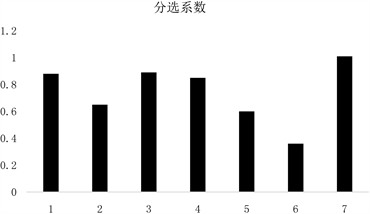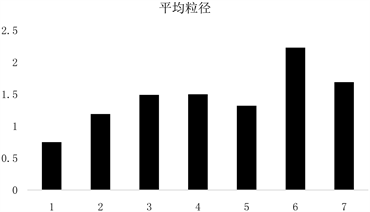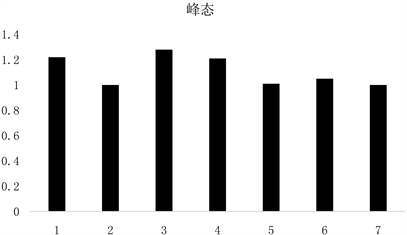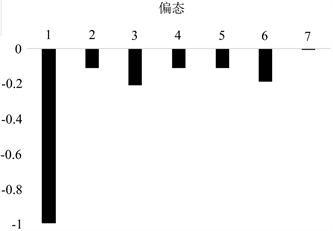(其中从1~7分别是：日照市万平口海水浴场、日照市山区虎山镇海滩、日照市东港区秦楼街道富蓉村海滩、日照市东小庄村位于日照市东港区、日照市东港区秦楼街道大陈家村海滩、日照市东港区两城镇肖家村海滩、日照市东港区涛雒镇海滩。)

Figure 3. Comparison of sediment particle size parameters of each beach

4.2. 研究区海滩地貌分析Table 3. Rizhao City coastal zone wave characteristics

$\epsilon =\frac{{a}_{b}{\omega }^{2}}{g{\mathrm{tan}}^{2}\beta }$$\omega =2\text{π}/T$

${H}_{b}=\frac{0.563{H}_{0}}{\left(\frac{{H}_{0}}{{L}_{0}}\right)}$

${L}_{0}$ 为平均波长，深水波长按照海港水文规范提供的公式计算： ${L}_{0}\cong 1.17{\stackrel{¯}{T}}^{2}$ 。根据上述公式计算得各海滩数据值如表4所示。

4.3. 海滩保护建议

1) 宣传环境保护的观念，提高公众的环保意识

2) 研究海滩的形成和及其演变规律。

3) 优化产业结构，获得经济环境齐发展

4) 完善法律法规，提高公众意识

5) 加强监管，提高政府办事效率

5. 结论

1) 日照市海岸带地区多数调查海滩的平均粒径在0.7~2.5 φ之间，总体上海滩的分选性为中等或者相对较差，其粒度频率曲线尖锐，峰态以窄态分布为主，偏态主要是负偏状态，物质来源较为复杂，而重点研究区域即日照市东港区万平口海水浴场的平均粒径为0.75 φ，以粗砂为主，其粒度频率曲线也是相对尖锐，峰态以窄态分布为主，偏态主要是呈现负偏状态；

2) 根据构造成因和地貌特征及其研究区海滩特征，认为日照市海岸带的海滩类型主要为沙坝–泻湖型和夷直型两种，并且各研究区的耗散值都相对较高，均为耗散型海滩， $\epsilon$ 值均大于20，而研究的重点区域万平口海水浴场为沙坝–泻湖型，其 $\epsilon$ 值相对偏小一些，物质来源相对比较复杂；

3) 研究得到日照市海岸带的地貌特征与其水动力过程即波浪、潮汐、河流等因素密切相关，日照海岸带地貌特征的形成与分布受到的潮汐作用更为强烈，K均 < 1，水动力过程以潮汐作用占据主导地位，同时人类活动和地质构造也会对海岸带地貌特征造成重要影响。

 刘建辉. 福建砂质海岸侵蚀机制及影响因素分析[D]: [博士学位论文]. 青岛: 中国海洋大学, 2010.

 杨敏. 日照市海洋旅游竞争力研究[D]: [硕士学位论文]. 青岛: 中国海洋大学, 2009.

 佘运勇, 王剑, 王艳华, 等. 南黄海海洋表层沉积物中重金属的分布特征及潜在生态风险评价[J]. 海洋环境科学, 2011, 30(5): 631-635.

 夏鹏, 臧家业, 王湘芹. 连云港近岸海域表层沉积物中重金属的地球化学特征及其源解析[J]. 海洋环境科学, 2011, 30(4): 520-524.

 夏东兴, 王文海. 中国海岸侵蚀述要[J]. 地理学报, 1993(5): 468-476.

 盛静芬, 朱大奎. 海岸侵蚀和海岸线管理的初步研究[J]. 海洋通报, 2002, 21(4): 50-57.

 褚智慧. 日照市海滩沉积地貌及质量评价[D]: [硕士学位论文]. 青岛: 中国海洋大学, 2013.

 王松涛, 吴振, 衣伟虹, 等. 日照市海岸带地质环境质量评价研究[J]. 山东国土资源, 2014(12): 54-58.

 蔡锋. 华南沙质海滩动力地貌过程[D]: [博士学位论文]. 青岛: 中国海洋大学, 2005.

 Friedman, G.M. (1979) Address of the Retiring President of the International Asso-ciation of Sedimentologists: Differences in Size Distributions of Populations of Particles among Sands of Various Ori-gins. Sedimentology, 26, 3-32.
https://doi.org/10.1111/j.1365-3091.1979.tb00336.x

 金秉福. 粒度分析中偏度系数的影响因素及其意义[J]. 海洋科学, 2012, 36(2): 129-135.

 Guza, R.T. and Inman, D.L. (1975) Edge Waves and Beach Cusps. Journal of Geophysical Research, 80, 2997-3012.
https://doi.org/10.1029/JC080i021p02997

 李善为, 夏东兴. 山东海岸地貌发育特征[J]. 海洋湖沼通报, 1981(3): 41-47.

 崔金瑞, 夏东兴. 山东半岛海岸地貌与波浪、潮汐特征的关系[J]. 海洋科学进展, 1992(3): 20-25.

Top